﻿ 基于神经网络精准规划船舶航行路线的算法
 舰船科学技术2022, Vol. 44Issue (10): 143-146    DOI: 10.3404/j.issn.1672-7649.2022.10.030PDF

Research on algorithm of accurately planning ship route based on neural network
CAO Shi-yong
Jiangsu Shipping College, Institute of Navigation Technology, Nantong 226001, China
Abstract: Accurate planning of navigation route and reasonable avoidance of obstacles are the key to safe operation. Therefore, an algorithm for accurately planning ship route based on neural network is designed. The weight of neural network is optimized by genetic algorithm, and the ship track prediction model based on neural network is established. The ship course and speed are input into the model, and the ship longitude and latitude difference, that is, the ship track prediction result, is output. According to the result, the route planning environment during ship navigation is obtained; According to the Markov decision-making process, the optimal strategy network of route planning is obtained, the optimal strategy is determined through the deep reinforcement learning neural network, the prediction results of route planning environment are input into the network, the ship execution actions within the planning strategy are determined, and the navigation route is accurately planned. Experiments show that the algorithm can accurately predict the ship track. In different waters and scenarios, the navigation route can be accurately planned to ensure that there is no collision risk during navigation and reduce the average distance deviation of track points.
Key words: neural network     precise planning     navigation route of the ship     genetic algorithm     Markov     reinforcement learning
0 引　言

1 精准规划船舶航行路线算法 1.1 基于神经网络的船舶航迹提取 1.1.1 基于神经网络的船舶航迹预测模型

 ${g_j} = \frac{{\displaystyle\sum\limits_{i = 1}^2 {{w_{ij}}{x_i}} }}{{1 + {e^{ - x}}}} 。$ (1)

 ${y_k} = \frac{{\displaystyle\sum\limits_{j = 1}^\varpi {{w_{jk}}{g_j}} }}{{1 + {e^{ - x}}}} ，$ (2)

 ${w_{jk}} = {w_{jk}} + \eta \frac{{\displaystyle\sum\limits_n {{w_{jk}}{g_n}} }}{{1 + {e^{ - x}}}}\left( {{t_k} - {y_k}} \right){g_j} 。$ (3)

 ${w_{ij}} = {w_{ij}} + \eta \frac{{\displaystyle\sum\limits_m {{w_{mj}}{x_m}} }}{{1 + {e^{ - x}}}}\left[ {\sum\limits_n {{y_n}\left( {1 - {y_n}} \right)\left( {{t_n} - {y_n}} \right){w_{jn}}} } \right]{x_i}。$ (4)

1.1.2 基于GA优化的船舶航迹预测模型

BP神经网络预测船舶航迹时，权值影响航迹预测精度，通过GA优化BP神经网络的权值，提升船舶航迹预测精度，优化流程如图1所示。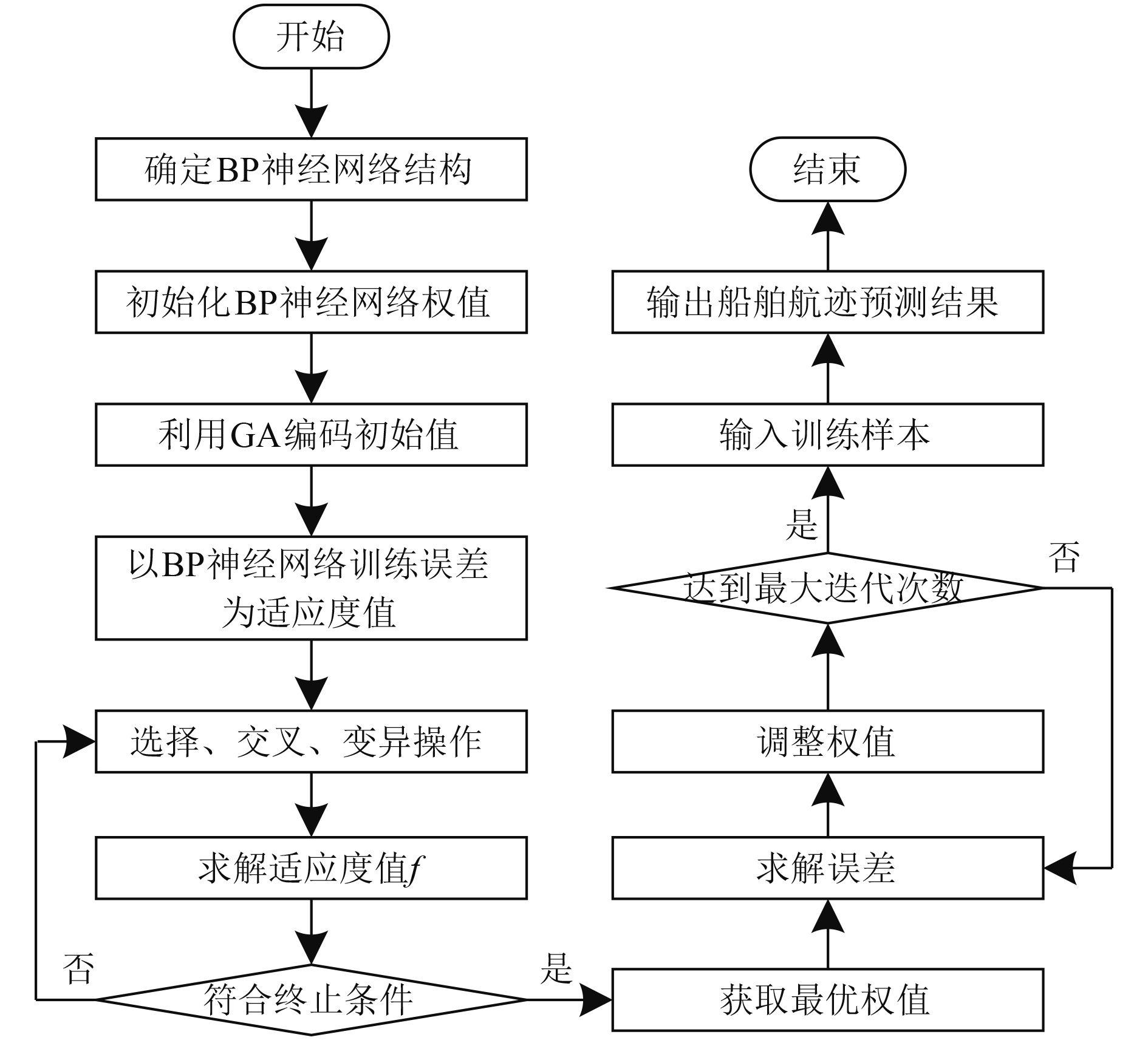图 1 GA优化BP神经网络流程图 Fig. 1 Flow chart of GA optimized BP neural network

1.2 精准规划船舶航行路线算法设计 1.2.1 马尔可夫的船舶航行路线规划的决策过程

MDP的动态过程是智能体即船舶的初始状态为 ${h_0}$ ，在B内选择一个 ${b_0}$ 并执行，依据 $P_{hh'}^b$ 将智能体转移至下一个 ${h_1}$ 内，再执行 ${b_1}$ ${h_1}$ 转移至 ${h_2}$ ，然后执行 ${b_2}$ ，反复操作，以全部动作执行完毕为止。每次动作执行后，船舶航行环境均会为当下动作给出立即奖励r

 ${Z^{\text{π}} }\left( h \right) = {E_{\text{π}} }\left[ {\mu {r_{\alpha + 1}} + \gamma \sum\limits_{k' = 0}^\infty {{\gamma ^{k'}}\mu {r_{\alpha + k' + 2}}\left| {{h_\alpha } = h} \right.} } \right]，$ (5)

 ${Q^{\text{π}} }\left( {h,b} \right) = {E_{\text{π}} }\left[ {\sum\limits_{k' = 0}^\infty {{\gamma ^{k'}}\mu {r_{\alpha + k' + 1}}\left| {{h_\alpha } = h,{b_\alpha } = b} \right.} } \right]。$ (6)

1.2.2 精准规划船舶航行路线算法优化

α时刻，船舶通过分析当下的其余船舶航迹预测结果，得到该船舶的航行环境状态 ${H_\alpha }$ ，执行动作 ${b_\alpha }$ ，再得到一个期望奖励 ${r_\alpha }$ ，令 ${R_\alpha } = \displaystyle\sum\limits_{k' = 0}^\infty {{\gamma ^{k'}}\mu {r_{\alpha + k' + 1}}}$ 。船舶在航行路线规划策略π内，选取需执行的动作，当下π内H执行b能够得到的回报是 ${Q^{\text{π}} }\left( {h,b} \right)$ ，当下π内H可得到的回报期望是 ${Z^{\text{π}} }\left( H \right)$ ，公式如下：

 $\begin{gathered} {Z^{\text{π}} }\left( H \right) = {E_{a \sim {\text{π}} \left( h \right)}}\left[ {{Q^{\text{π}} }\left( {h,b} \right)} \right] ，\hfill \\ {Q^{\text{π}} }\left( {h,b} \right) = E\left[ {{R_\alpha }\left| {{h_\alpha } = h,{b_\alpha } = b,{\text{π}} } \right.} \right] \hfill 。\\ \end{gathered}$ (7)

 ${Q^{\text{π}} }\left( {h,b} \right) = {E_{h'}}\left[ {\mu r + \gamma {E_{b' \sim {\text{π}} \left( h \right)}}\left[ {{Q^{\text{π}} }\left( {h',b'} \right)} \right]\left| {h,b,{\text{π}} } \right.} \right]。$ (8)

 ${Q^*}\left( {h,b} \right) = {E_{h'}}\left[ {\mu r + \gamma \mathop {\max }\limits_{b'} {Q^*}\left( {h',b'} \right)\left| {h,b} \right.} \right]。$ (9)

 $L\left( \theta \right) = {E_{h,b,r,h'}}\left[ {\left( {\mu r + \gamma \mathop {\max }\limits_{b'} Q\left( {h',b';\theta ,} \right)} \right) - Q\left( {h,b;\theta } \right)} \right] 。$ (10)

2 实验分析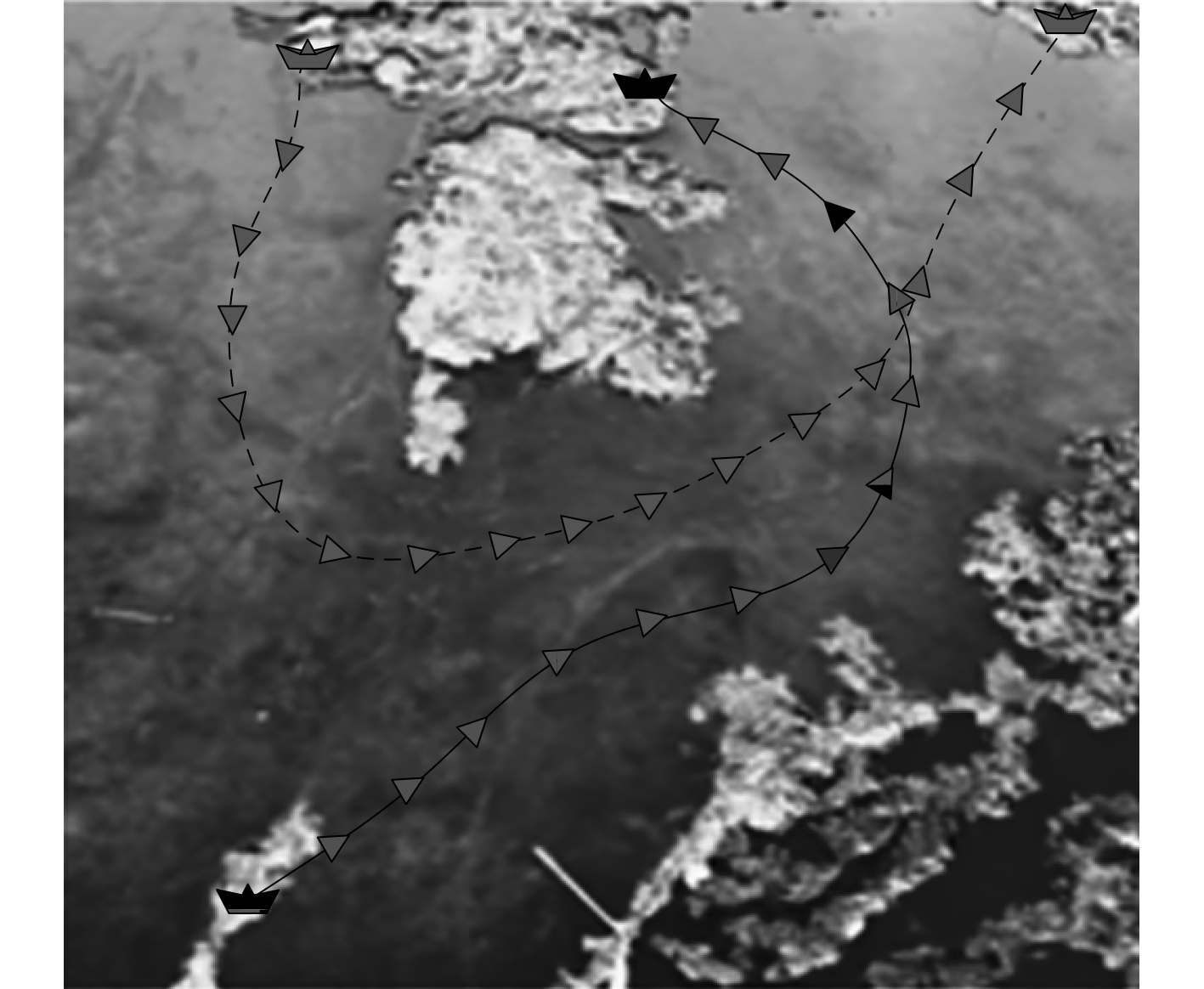图 2 两艘船的航迹预测结果 Fig. 2 Track prediction results of two ships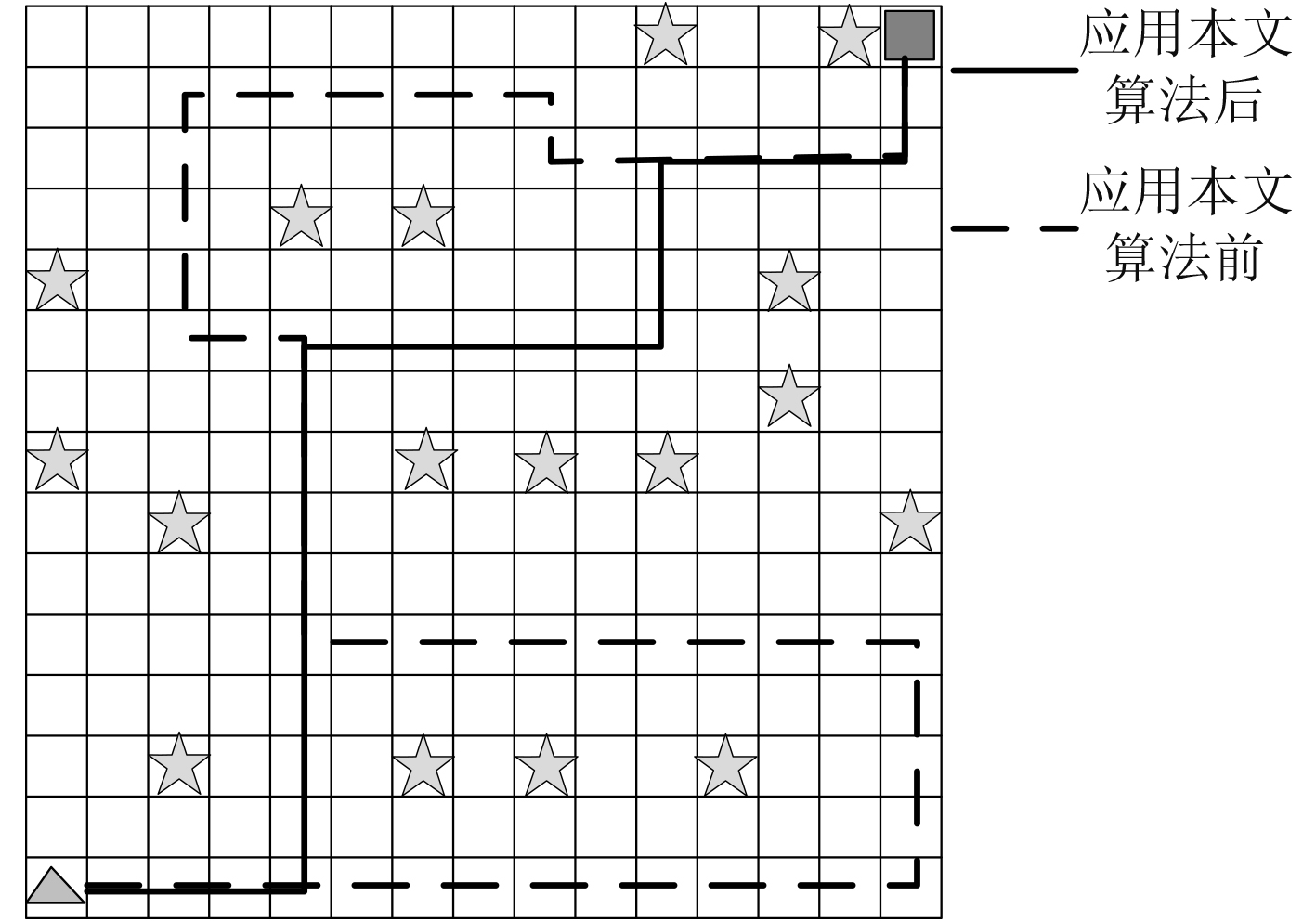图 3 航行路线规划结果 Fig. 3 Results of precise navigation route planning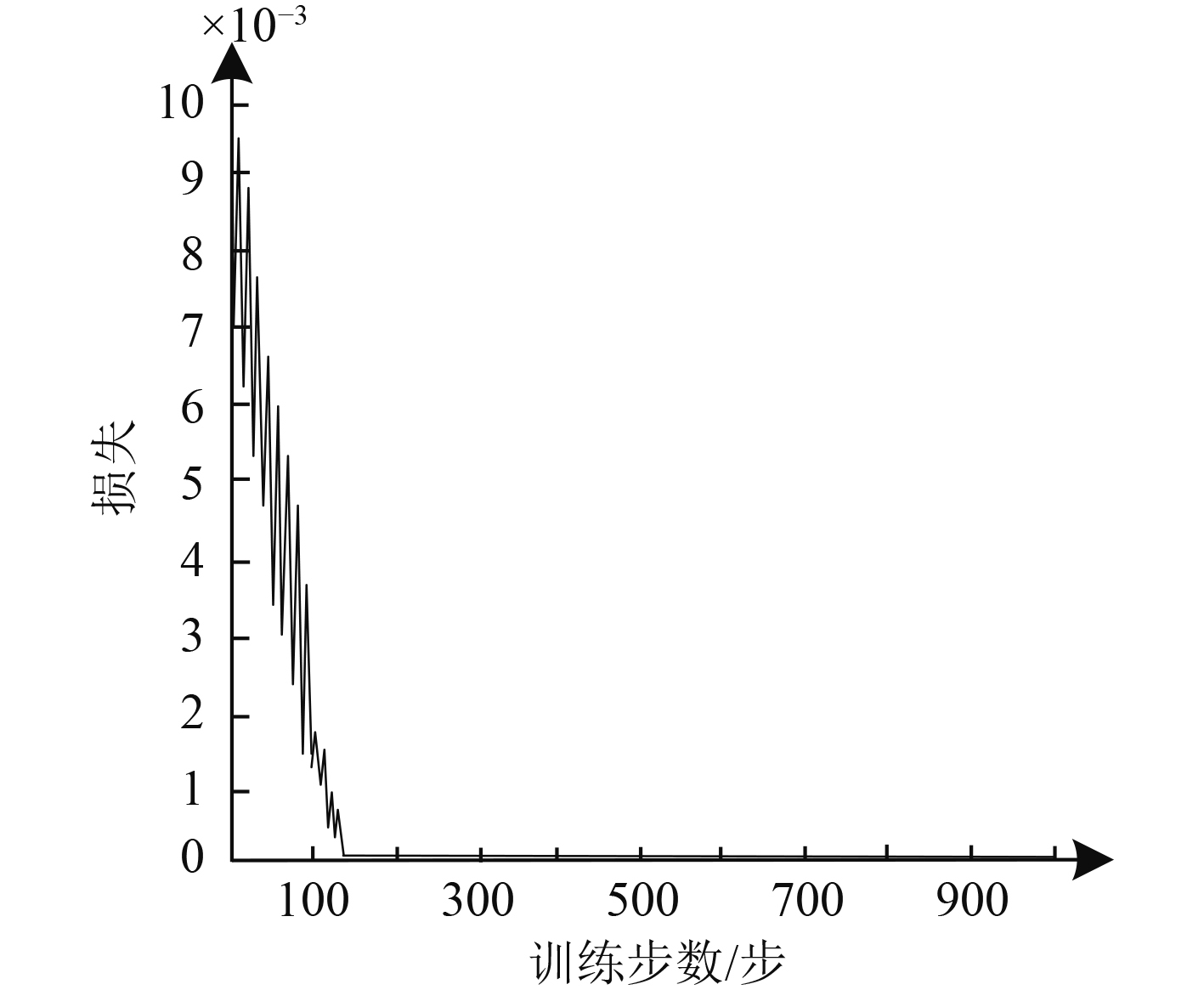图 4 训练步数和损失间的关系 Fig. 4 The relationship between training steps and losses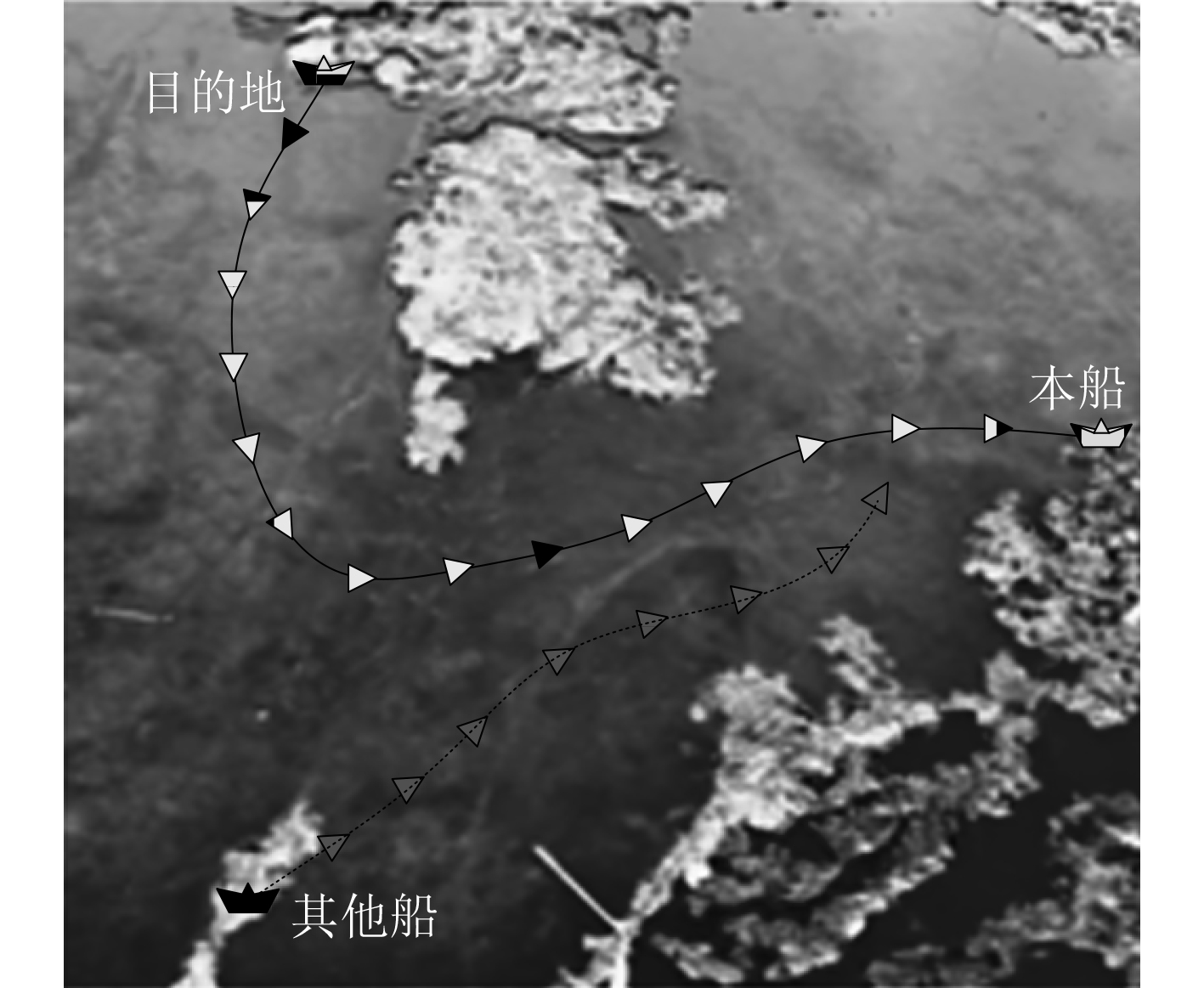图 5 会遇时船舶航行路线规划结果 Fig. 5 Result of the ships navigation route in planning when meeting表 1 航行路线规划效果 Tab.1 Effect of navigation route planning

3 结　语

  罗贤程, 尹建川, 李宏宾. 基于动态分阶势场法的船舶自动避碰系统[J]. 上海海事大学学报, 2020, 41(1): 1-7.  蒋仁炎, 俞万能, 廖卫强, 等. 智能全电船的低能耗路径规划算法研究[J]. 中国造船, 2021, 62(2): 245-254. DOI:10.3969/j.issn.1000-4882.2021.02.022  唐皇, 魏立桥, 尹勇, 等. 基于概率有向图模型的船舶进出港航路规划[J]. 华中科技大学学报(自然科学版), 2020, 48(5): 105-109.  潘伟, 董其林, 许小卫, 等. 考虑通航环境因素的智能船舶航线规划[J]. 上海海事大学学报, 2021, 42(3): 76-84.  曹旺, 邓烈威, 郭瑶, 等. 基于改进NSGA-Ⅲ的船舶路径规划方法[J]. 船舶工程, 2021, 43(S1): 343-347+375.  倪生科, 刘正江, 蔡垚, 等. 基于混合遗传算法的船舶避碰路径规划[J]. 上海海事大学学报, 2019, 40(1): 21-26.  胡玉可, 夏维, 胡笑旋, 等. 基于循环神经网络的船舶航迹预测[J]. 系统工程与电子技术, 2020, 42(4): 871-877.  祁林, 渠俊锋, 司文杰, 等. 基于RBF神经网络的水面船舶轨迹跟踪控制[J]. 船舶工程, 2021, 43(1): 95-101+118.  童帮裕, 胡坚堃. 基于改进蚁群算法的船舶冰区航行路径规划[J]. 中国航海, 2020, 43(1): 24-28. DOI:10.3969/j.issn.1000-4653.2020.01.005  王立鹏, 张智, 马山, 等. 考虑船舶操纵性约束的改进遗传算法航线规划[J]. 哈尔滨工程大学学报, 2021, 42(7): 1056-1062.  孟顺, 郭俊林, 刘志强, 等. 基于船舶火灾蔓延态势的路径规划算法[J]. 船舶工程, 2021, 43(2): 110-115.  吕进锋, 马建伟, 李晓静. 基于改进的随机路径图及和声算法的舰船航线规划[J]. 控制理论与应用, 2020, 37(12): 2551-2559.  陈新, 袁宇浩, 饶丹. 一种改进A~*算法在无人船路径规划中的应用[J]. 计算机仿真, 2021, 38(3): 277-281. DOI:10.3969/j.issn.1006-9348.2021.03.057